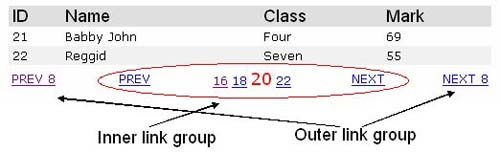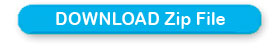# Maximum page jump in advance paging script

You must read how the basic paging script works before learning on this advanced script. The advanced script is different in only how the bottom navigational system works. Here some key points.

The basic script for displaying records is same.

There are two variables which are to be passed to each page. In basic script this is one only. Read the URL like this

In basic script

### https://www.plus2net.com/php_tutorial/demo_paging1.php?start=20

In advanced script it is

We are sending one extra variable in advanced script. However based on the value of the variable start the records are displayed. But here bottom links records are not displayed till the end, it only display links up to the next higher group. You can see link groups are created in two levels, one link group is higher group or outer one and one is within group or inner link group.Inside the script we set two variables, one is number of records per page that is our \$limit value and now one more \$p_f value we have to set in advance script. The value of \$p_f is the jump for outer link group. In our example we have seen it is set the 8 and records per page that is \$limit is set to 2. Note that the value for \$p_f has to be always greater than the value of \$limit.

Now note that the links of the inner group has to show previous or next links and in between page links by staying within the larger group. The lower and upper limit of the inner group has to be within the boundary of outer group.

Now let us see how the navigational system of outer group is decided.

For the left most link the code is

### if(\$p_f<>0){print "<a href='\$page_name?start=\$p_back&p_f=\$p_back'><font face='Verdana' size='2'>PREV \$p_limit</font></a>"; }

This link will be visible if \$p_f value is not equal to zero , that mean this link will not be displayed at the starting. Now once this link is clicked it will carry the new value of \$p_f which is present value minus the jump value of outer group. This value of \$p_back is set like this before.

### \$p_back=\$p_f-\$p_limit;

In the same way the Next link of outer group is displayed. Here before the link the value of \$p_fwd is calculated like this.

### \$p_fwd=\$p_f+\$p_limit;

Now with this value of \$p_fwd there will be jump for the outer group links to next value. Here is the link

### print "<a href='\$page_name?start=\$p_fwd&p_f=\$p_fwd'><font face='Verdana' size='2'>NEXT \$p_limit</font></a>";

Now let us work for the inner link group. This group has to work within the start value and start value is equals to \$p_f value. For example if our \$p_f value is 16 then the inner link should start from 16. Now let us try for upper limit of inner link group, the upper limit has to satisfy two conditions. One is it should never goes up more than the total number of records that is the variable \$nume and it should be within the value of present \$p_f + the jump of outer link group. In our example we have set the jump for outer link group to 8. So if we are at 16th record then upper limit for inner group should be 16 + 8 = 24. What we have discussed here now is coded in this for loop.

### for(\$i=\$p_f;\$i < \$nume and \$i<(\$p_f+\$p_limit);\$i=\$i+\$limit){ }

We have left the internal linking structure inside the for loop as it is same as the basic script. Just read how the for loop in the above code is designed.

Hope this helps in understanding how the advance paging works.

Demo of Advance paging scriptVisitors Rating

Displaying records in multiple pages: PHP MySQL Paging

Post your comments , suggestion , error , requirements etc here .